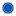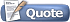View Single Post09-10-2015, 07:47 AM   #1
sickufully
Sir KriskJoin Date: Dec 2007
Location: Vic, Australia
Posts: 928[University - C++ Programming] Filling an Array with RNG & Display Contents

I'm back again with some more C++ questions. This time it's a little easier, it's as simple as filling an array with 20 random numbers and then displaying them to the screen. Most of the code has been provided by my teacher. I'm pretty sure I can get the RNG to work, I'm just getting a few confusing errors.

This is the exercise:
Quote:
 For this task you are required to create an array of 20 integers, which you will populate with a random number with a value between 1 and 20. Once you have created this array of numbers, you will then need to go through and display the value of each of the array elements.
Here is my code so far:
Code:
```#include <iostream>
#include <cstdlib>
#include <ctime>
#include <time.h>
#include <stdlib.h>

using namespace std;
using std::srand;
using std::rand;
using std::time;

int randArray;
int i;

void Display(int *, int);
int main(void)
{

int randArray;

srand(time(NULL));

for (int i = 0; i < 20; i++) {
randArray[i] = rand() % 20 + 1;

// The following 4 lines of code were to check the values entered into array elements. I will remove it when I'm confident that the values are correct.
cout << randArray << endl;
cout << randArray << endl;
cout << randArray << endl;
cout << randArray << endl;

Display(randArray, sizeof(randArray) / sizeof(int));
return 0;
}
void Display(int *arrayData, int numElements)
{
for (int counter = 0; counter < numElements; counter++)
{
std::cout << *(arrayData + counter) << std::endl;
}
return;
}
}```
My first error is the fact that the only number being entered into the array is -883XXXXXX. Basically some huge random number and I don't understand where it's coming from.

My second error is the fact that I don't understand anything beyond the line starting with Display(randArray, sizeof(.....) . If someone could explain this to me in the most simple possible way, I'd very much appreciate it.

As for the third error, well there isn't one yet but I'm sure some problem will arise.

If you could tell me what's correct, what needs tweaking and what is completely wrong, it should hopefully guide me in the right direction. Again, I'm willing to pay credits for relevant help, just let me know if you want any once I'm done with the exercise.

Thanks for any help in advance.
__________________